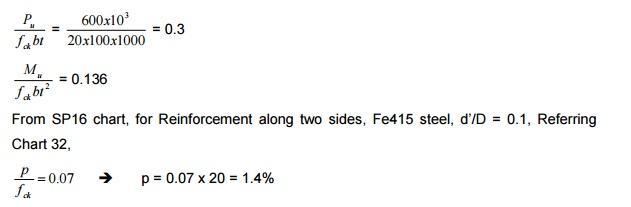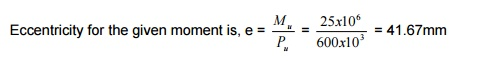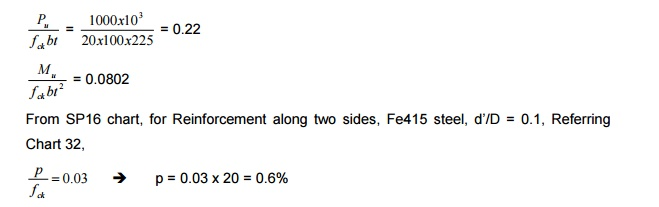Home | | Design of Reinforced Concrete and Brick Masonry Structures | Design Of Reinforced Concrete Wall

# Design Of Reinforced Concrete Wall

Classified as o plain concrete wall, when rein. < 0.4% o Reinforced concrete wall, when rein. > 0.4%

DESIGN OF REINFORCED CONCRETE WALL

-      Compression member

-      In case where beam is not provided and load from the slab is heavy

-      When the masonry wall thickness is restricted

-      Classified as

plain concrete wall, when rein. < 0.4%

Reinforced concrete wall, when rein. > 0.4%

Load from slab is transferred as axial load to wall. When depth is large, it is called RC wall. Design is similar to a RC column, breadth equal to thickness of wall and depth equal to 1m.

-      Axially loaded with uniaxial bending

General conditions:

Classification of concrete walls:

1.   Plain concrete wall

2.   Reinforced concrete wall

In plain concrete wall, the reinforcement provided is less than 0.4% of c/s. In reinforced concrete wall, the percentage of steel provided is greater than 0.4% and is designed similar to reinforced concrete columns. Slenderness ratio is equal to

Least of (l/t or h/t), where,

l effective length of wall,   h effective height of wall,  t thickness of wall

If       ?   <   12,   the   wall   is   short   and   if   ?   >   12,   t

Braced and Unbraced:

Braced : When cross walls are provided for the walls such that they can take lateral load and 2.5% of vertical load, then the wall is braced. Otherwise, the wall is known as unbraced wall.

Note: Other walls under special cases are,

i)             Cantilever wall

Shear walls -To take lateral loads [Take care of flexure developed due to lateral loading on the structure, depth is provided along the transverse direction]

Guidelines for RC wall:

1.      The limiting   slendernesslim)ifanyforunbraced(?wall is 30 and for braced wall is           45.

2.      For   short   bracedu =RC0.4.f ckwall.Ac+0.67 .f(?y.Ast    <   12),   P

3.   For short unbraced RC wall, along with the above axial load Pu, the moment due to minimum eccentricity is checked for emin = t/20 or 20mm, where, M = P x e.

For the above axial load and moment, the RC wall is designed similar to RC column subjected to axial load and uniaxial moment.

4.   Slender   braced   wall   (?   <   45):

The additional moment due to additional eccentricity as per Table 1 of SP16 is considered. Where the additional eccentricity,The additional moment due to eccentricity is added with the moment on the column and moment on the wall. The wall is designed for axial load with uniaxial moment.

5.   For slender unbraced wall Similar procedure[?limitedasincase4 isto 30 adopted.

6.   Detailing of reinforcement [IS456 Guidelines]:

a.   For plain concrete wall, minimum vertical steel is 0.12% for HYSD bars and 0.15% for mild steel bar

b.   For RC wall, minimum vertical reinforcement is 0.4% of c/s

c.   In plain concrete wall, transverse steel is not required

d.   In RC wall, transverse steel is not required (not less than 0.4%)

e.   Maximum spacing of bars is 450mm or 3t, whichever lesser

f.     The thickness of wall in no case should be less than 100mm

g.   If thickness is greater than 200mm, double grid reinforcement is provided along both the faces.

7.   BS 8110 guidelines:

a.   Horizontal reinforcement same as IS456

b.   Vertical reinforcement not to be greater than 4%

When compression steel is greater than 2% of vertical reinforcement, horizontal reinforcement of 0.25% for HYSD bars or 0.3% of MS bars are provided. [As per IS456, it is 0.2% for HYSD bars and 0.3% for mild steel bars].

d.   The diameter of transverse bars (horizontal) should not be less than 6mm or ?L/4.

8.   Links are provided when the compression steel is greater than 2%. Horizontal links are provided for thickness less than 220mm. Diagonal links are provided when

thickness is greater than 220mm. The spacing of links should be less than 2t and diameter of links Lnot/4. less than 6mm or ?/41) Design a reinforced concrete wall 3m height, 4m length between cross walls. The wall is 100mm thick and carries a factored load of 600 kN/m length. Use M20 concrete and Fe415 steel.

Since cross walls are provided, the wall is braced.

?             = h/t or l/t

= 3000/120 or 4000/120

= 30 or 40

Assume both ends fixed (restrained against rotation and displacement)

Leff    = 0.75 lo     where, lo is least of length and height

= 0.75 x 3 = 2.25m

?        =   2250/100   =   22.5   >  12

?lim = 45 > 22.5 [? lim<] ? Accidental minimum eccentricity due to,

emin = t/20 or 20mm = 5 or 20mm

Therefore, moment due to accidental eccentricity of 20mm is considered. Additional eccentricity due to slenderness,Total eccentricity = emin + ea = 20 + 25.3 = 45.3mm

Moment Mu = Pu x 45.3 = 27.2 x 106 Nmm

For the axial load and moment, RC wall is designed similar to a RC column subjected to axial load and uniaxial moment.Area of steel = 1.4/100 x 100 x 1000 = 1400 mm2

Provide 16mm @ 140mm c/c as vertical compression bar

Horizontal -Provide a nominal transverse reinforcement of 0.4% of c/s Ast = 0.4/100 x 1000 x 100 = 400 mm2

Provide 8mm @ 120mm c/c

Since vertical reinforcement is less than 2%, no horizontal links are required.

2) A reinforced concrete wall of height 5m is restrained in position and direction carrying a factored load of 600 kN and factored moment of 25kNm at right angles to the plane of the wall. Use M20 concrete and Fe415 steel. Design the wall.The eccentricity is compared with emin. The larger of the two is added with additional eccentricity due to slenderness, if any.

Assume l/d of 22. [Generally assume l/d from 20 -25] d = 5000/22 = 227.27

Assume a thickness of 225mm Leff = 0.75 x 5 = 3.75m

?act = 3750/225 = 16.67 > 12 The given wall is slender. emin = t/20 or 20mm

= 225/20 or 20mm

11.25mm or 20mm < 41.67mm Additional eccentricity due to slenderness,Total eccentricity = emin + ea = 41.67 + 31.25 = 72.92mm

Moment Mu = Pu x 72.92 = 600 x 1000 x 72.92 = 43.75 x 106 Nmm

For the axial load and moment, RC wall is designed similar to a RC column subjected to axial load and uniaxial moment.Area of steel = 0.4/100 x 225 x 1000 = 900 mm2

Provide 12mm @ 120mm c/c as vertical compression bar

Since thickness of wall is 225mm, reinforcement is provided on both faces of the wall. Therefore, provide 12mm @ 250mm c/c < 3t and 450mm

Horizontal -Provide a nominal transverse reinforcement of 0.4% of c/s on both faces. Ast = 0.4/100 x 1000 x 100 = 400 mm2

Provide 8mm @ 120mm c/c

Since vertical reinforcement is less than 2%, no horizontal links are required.

3) In the above problem, design the wall for factored axial load of 1000kN and factored moment of 50kNm.

Pu = 1000kN, Mu = 50kNm

e = 50 x 106/1000 x 103 = 50mm

l/d = 22   [Generally l/d taken from 20 -25]

d = 5000/22 = 227.27mm

Adopt a thickness of 225mm. leff = 0.75 x 5000 = 3750mm ?act = 3750/225 = 16.67 > 12 ?min = 45 > 16.67

Wall is slender.

Emin = t/20 or 20mm

= 225/20 or 20mm

= 11.25mm or 20mm < 41.67mmTotal eccentricity = emin + ea = 50 + 31.25 = 81.25mm

Moment Mu = Pu x 81.25 = 1000 x 1000 x 81.25 = 81.25 x 106 Nmm

For the axial load and moment, RC wall is designed similar to a RC column subjected to axial load and uniaxial moment.Area of steel = 0.6/100 x 225 x 1000 = 1350 mm2

Provide 16mm @ 140mm c/c as vertical compression bar

Horizontal -Provide a nominal transverse reinforcement of 0.4% of c/s Area of steel = 0.4/100 x 225 x 1000 = 900 mm2

Since thickness of wall is 225mm, reinforcement is provided on both faces of the wall. Therefore, provide 12mm @ 250mm c/c < 3t and 450mm

Since vertical reinforcement is less than 2%, no horizontal links are required.

Study Material, Lecturing Notes, Assignment, Reference, Wiki description explanation, brief detail
Civil : Design Of Reinforced Concrete And Brick Masonry Structures- Design Of Reinforced Concrete Wall : Design Of Reinforced Concrete Wall |